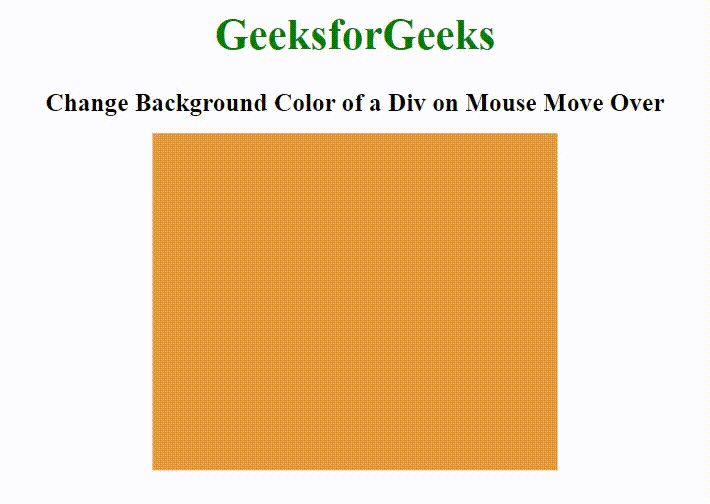GeeksforGeeks App
Open AppBrowser
Continue

# How to Change Background Color of a Div on Mouse Move Over using JavaScript ?

The background color of the div box can be easily changed using HTML, CSS, and Javascript. We will use the querySelector() and addEventListener() methods to select the element and then apply some math logic to change its background color. The below sections will guide you on how to create the effect.

HTML Code: In this section, we will create a basic structure of the body. The body section contains an <div> element whose background color will be changed when the mouse moves over the div element.

Example: In this example, we will see the change of background color while mouse move over.

## HTML

 ```<``html` `lang``=``"en"` `dir``=``"ltr"``>` `<``head``>``    ``<``meta` `charset``=``"utf-8"``>``    ``<``title``>``        ``How to Change Background Color``        ``of a Div on Mouse Move Over``        ``using JavaScript ?``    ````` `<``body``>``    ``<``div` `class``=``"first"``>``` ``

CSS Code: In this section, we will use some CSS property to style the div element.

## CSS

 `.first {``    ``position``: ``absolute``;``    ``background``: ``#E73C49``;``    ``width``: ``300px``;``    ``height``: ``250px``;``    ``top``: ``50%``;``    ``left``: ``50%``;``    ``transform: translate(``-50%``, ``-50%``);``}`

Javascript Code:

Step 1: The first step is to create an array consisting of different colours.

Step 2: The second step is to use the querySelector() method to select the div element and then use addEvenListener() method to attach an event handler (mouseover) to it.

Step 3: In the last step, we will style the background of the div element using some logic i.e. we will use Math.random() function on the array to return a floating-point number between the array range then use Math.floor() method to round the floating number downward to its nearest integer.

## JavaScript

 `var` `color = [, ``"#3C9EE7"``, ``"#E7993C"``,``            ``"#E73C99"``, ``"#3CE746"``, ``"#E7993C"``];` `document.querySelector(``"div"``).addEventListener(``    ``"mouseover"``, ``function` `() {` `        ``document.querySelector(``"div"``).style.background``            ``= color[(Math.floor(Math.random() * color.length))];``    ``})`

Complete Code: In this section, we will combine the above three code sections.

## HTML

 ```<``html``>` `<``head``>``    ``<``meta` `charset``=``"utf-8"``>``    ``<``title``>``        ``How to Change Background Color``        ``of a Div on Mouse Move Over``        ``using JavaScript ?``    ``` `    ``<``style``>``        ``.first {``            ``position: absolute;``            ``background: #E73C49;``            ``width: 300px;``            ``height: 250px;``            ``top: 50%;``            ``left: 50%;``            ``transform: translate(-50%, -50%);``        ``}``    ````` `<``body``>``    ``<``div` `class``=``"first"``>` `    ``<``script` `type``=``"text/javascript"``>``        ``var color = [, "#3C9EE7", "#E7993C",``            ``"#E73C99", "#3CE746", "#E7993C"];` `        ``document.querySelector("div").addEventListener(``            ``"mouseover", function () {` `                ``document.querySelector("div").style.background``                    ``= color[(Math.floor(Math.random() * color.length))];``            ``})``    ````` ``

Output:My Personal Notes arrow_drop_up
Related Tutorials